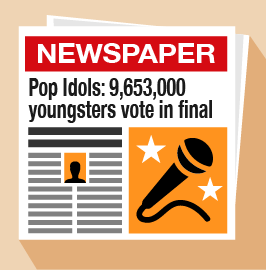Science, Maths & Technology

### Become an OU studentEveryday maths 1

Start this free course now. Just create an account and sign in. Enrol and complete the course for a free statement of participation or digital badge if available.

# 1.3 Working with whole numbers

The following activities cover everything in the whole numbers section. As you attempt the activities, look for key words to identify what the question is asking you to do.

## Activity 3: Looking at numbers

1. Look at this newspaper headline:• a.Write down the number in millions.
• b.Write down the number in thousands.
• c.Look at the details below. Who won the Pop Idols competition?
1. Look at the data in the following table. It gives the temperatures of five cities on a Monday in January.
City Temperature
London 0°C
Paris –1°C
Delhi 28°C
Moscow –10°C
•

• a.Which city was the coldest?
• b.Which city was the warmest?
• c.How many cities have a temperature below 5°C?
1. You buy a jumper for £24 and a skirt for £18. How much do you spend altogether?
1. You have £48. You spend £26. How much do you have left?

1. The answers are as follows:
• a.9 million
• b.653 thousand
• c.Will
2. The answers are as follows:
• a.Moscow
• b.Delhi
• c.London, Paris and Moscow
3. £24 + £18 = £42
4. £48 – £26 = £22

## Activity 4: Using multiplication and division

You can use a calculator in this activity.

1. What are the answers to these sums?
• a.6 × 4
• b.3 × 9
• c.5 × 7
• d.36 ÷ 9
• e.48 ÷ 6
• f.15 ÷ 3
2. Wine glasses come in boxes of 10. There are 25 boxes in a crate. How many wine glasses are there in one crate?
3. A circus is selling tickets at £19 for adults and £11 for children. How much would it cost for two adults and two children to go?

1. The answers are as follows:
• a.6 ×4 = 24
• b.3 × 9 = 27
• c.5 × 7 = 35
• d.36 ÷ 9 = 4
• e.48 ÷ 6 = 8
• f.15 ÷ 3 = 5
2. 10 × 25 = 250 wine glasses.
3. 2 × 19 = £38

11 × 2 = £22

22 + 38 = £60

It would cost £60 to go to the circus.